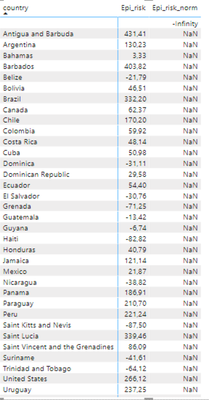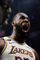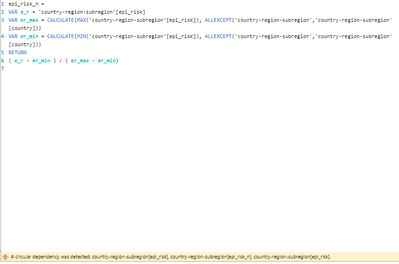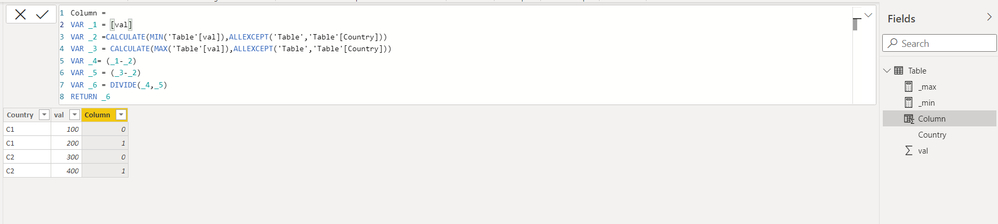cancel
Showing results for
Did you mean:Frequent Visitor

## New calculated column with the max of another calculated column

Hi,

I'd appreciate your help a lot. I'm stuck with a problem I thought I could solve using measures instead of columns (I solved what I thought were similar problems doing so), but nothing worked so far, and I really need it. I have a table of countries with many columns; one of them is a calculated column called epi_risk made with other tables' measures and being updated from source every day. Here a capture of just the countries and that column:I need to standardize the Epi_risk distribution to a 0-10 scale. For that, I have tried this:

epi_risk_norm =
VAR max_er = MAX('country-region-subregion'[epi_risk])
VAR min_er = MIN('country-region-subregion'[epi_risk])
VAR e_r_n = ([epi_risk_m] - min_er) / (max_er -min_er)
RETURN
e_r_n

But it works neither as a new calculated column nor new measure because the calculation iterates through all the rows, so (max_er - min_er) always is 0. How can I do it? Sure it will be a way to do it for but just starting with DAX, and I didn't find in the documentation a simple way.

Pedro

5 REPLIES 5Super User

Hi,

Regards,
Ashish Mathur
http://www.ashishmathur.comFrequent Visitor

Hi @Ashish_Mathur , thank you very much for your interest. Sorry if I didn't make myself clear. I'll try to explain better as, unfortunately, I cannot share the PBI file because my organization doesn't authorize me to do it because it's internal for now.

I have a table like the above with a column epi_risk (and with more columns and overall many other measures and calculated columns):

Table

country    epi_risk     epi_risk_n
A                16            0,882012724
B                23,45       0,98973395
C                -32,78      0,176691729
D                24,16        1
E                  -9,8         0,508964719
F                   -45           0
G                  7,7           0,762001157

I want to get the column epi_risk_n as this calculation:

country(i) - min(epi_risk) / max(epi_risk) -min(epi_risk) , so for the first row, country A, the value comes from (16-(-45)) / (24,16 - (-45)) = 0,8820. This is because I need to standardize the epi_risk in a scale from 0 to 10.

But I have failed till now. I could create neither a new calculated column nor a new measure. As I said in the previous post, If I try to create a new column, I get a circular dependency error:I tried then a new measure (I don't know why or what is behind but for previous similar calculations when I got the same problem and I switched to a measure, it worked), But on this occasion, it didn't:I don't get any error, but the calculation is done for each row, so max and min are the same and equal to the epi_risk value (so not the max of the entire column and the min of the entire column), so max-min is 0, so I get NaN for the entire column due to the division by 0.

I think it's important to mention that in the formulas above, country-region-subregion[epi_risk] is a calculated column, and [epi_risk_m] is a measure, so I cannot use transform data because the columns don't appear there.

I don't know if it's clear now, sorry if not; I don't know what else I could say, and I'd appreciate a lot your help because I really do need the values on a scale of 0 to 10.

Thanks,

PedroCommunity Support

Hi @pjporrino,

What is the data type of column[epi_risk]?

Maybe it is not supported to get max or min value in the same row.

Best Regards,Frequent Visitor

Hi @smpa01 , thanks a lot for your help. It doesn't work because I get a circular dependency issue:I don't know if it's because the column epi_risk is a calculated column within the same table and with values from the same table. I got similar problems before, and I solved creating a measure instead of a column. I tried this:as a measure and [epi_risk_m] is the same as 'country-region-subregion'[epi_risk] but as a measure in the same table (I cannot use the column to define the variable e_r in a formula of a measure).

In this case, I don't get the error regarding circular dependency, but it doesn't work, again division by 0.

Any idea?

Thank you very much,

PedroSuper User

@pjporrino  can you try the following``````Column =
VAR _1 = [val]
VAR _2 =CALCULATE(MIN('Table'[val]),ALLEXCEPT('Table','Table'[Country]))
VAR _3 = CALCULATE(MAX('Table'[val]),ALLEXCEPT('Table','Table'[Country]))
VAR _4= (_1-_2)
VAR _5 = (_3-_2)
VAR _6 = DIVIDE(_4,_5)
RETURN _6``````

Proud to be a Super User!

New Animated Dashboard: Sales CalendarAnnouncements#### Exclusive opportunity for Women!

Join us for a free, hands-on Microsoft workshop led by women trainers for women where you will learn how to build a Dashboard in a Day!#### Power Platform Conference-Power BI and Fabric Sessions

Join us Oct 1 - 6 in Las Vegas for the Microsoft Power Platform Conference.Top Solution Authors
Top Kudoed Authors
Users online (3,721)